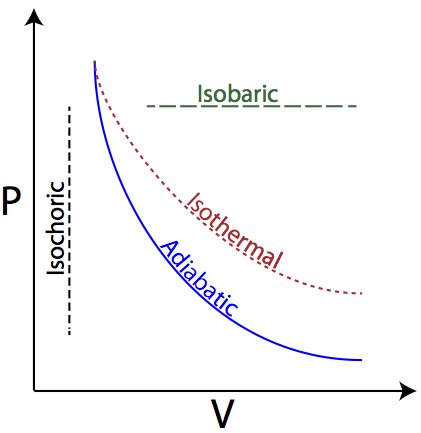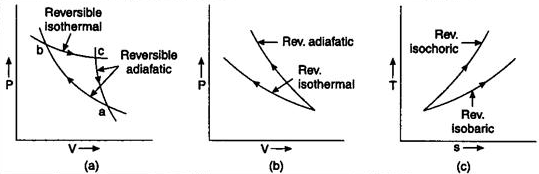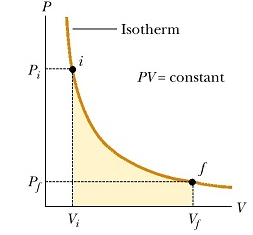# Please give list of all formulae of thermodynamics and suggest me the way to solve its numericals

2 years ago

• Kinetic Energy:- Energy possessed  by the atoms or molecules by virtue of their motion  is called kinetic energy.

• Internal Energy (ΔU):- Sum total of kinetic and potential energies of atoms/molecules constituting a system is called the internal energy of the system.

(a) ΔU is taken as positive if the internal energy of the system increases.

(b) ΔU is taken as negative if the internal energy of the system decreases.

• Heat:- Heat is the part of internal energy which is transferred from one body to another an account of the temperature difference.
• Work:- Work is said to be done when a force acting on a system displaces the body in its own direction.

dW = Fdx = PdV

W = P(V-Vi)

(a) If the gas expands, work is said to be done by the system. In this case V> Vi, therefore, W will be positive.

(b) If the gas is compressed, work is said to be done on the system. In this case Vf   Vi, therefore, work done is negative.

• Thermodynamic variables or parameters:- The thermodynamic state of system can be determined by quantities like temperature (T), volume (V), pressure (P), internal energy (U) etc. These quantities are known as thermodynamic variables, or the parameters of the system.

• Equation of state:- A relation between the values of any of the three thermodynamic variables for the system, is called its equation of state.

Equation of state for an ideal gas is PV = RT

• Equilibrium of a system:- A system is said to be in equilibrium if its macroscopic quantities do not change with time.

• Relation between joule and calorie:- 1 joule = 4.186 cal

• First law of thermodynamics:- If the quantity of heat supplied to a system is capable of doing work, then the quantity of heat absorbed by the system is equal to the sum of the increase in the internal energy of the system, and the external work done by it.

dQ = dU+dW

• Thermodynamic Process:- A process by which one or more parameters of thermodynamic system undergo a change is called a thermodynamic process or a thermodynamic change.(a) Isothermal process:- The process in which change in pressure and volume takes place at a constant temperature, is called a isothermal change. It may be noted that in such a change total amount of heat of the system does not remain constant.

(b) Isobaric process:- The process in which change in volume and temperature of a gas take place at a constant pressure is called an isobaric process.

(c) Isochoric process:- The process in which changes in pressure and temperature take place in such a way that the volume of the system remains constant, is called isochoric process.

(d) Adiabatic process:- The process in which change in pressure and volume and temperature takes place without any heat entering or leaving the system is called adiabatic change.

(e) Quasi-static process:- The process in which change in any of the parameters take place at such a slow speed that the values of P,V, and T can be taken to be, practically, constant, is called a quasi-static process.

(f) Cyclic process:- In a system in which the parameters acquire the original values, the process is called a cyclic process.

(g) Free expansion:- Such an expansion in which no external work is done and the total internal energy of the system remains constant is called free expansion.

• Reversible isothermal and adiabatic curve:-• Application of first law of thermodynamics:-

(a) Cooling caused in adiabatic process:- dT = PdV/Cv

(b) Melting:- dU = mLf

(c) Boiling:dU = mLv – P(V-Vi)

(d) Mayer’s formula:- CCv = R

• Specific heat capacity of gases:- Specific heat capacity of a substance is defined as the amount of heat required to raise the temperature of a unit mass of substance through 1ºC.

(a) Specific heat capacity at constant volume (cv):- Specific heat capacity at constant volume is defined as the amount of heat required to raise the temperature of 1 g of the gas through 1ºCkeeping volume of the gas constant.

Molar specific heat capacity, at constant volume (Cv), is defined as the amount of heat required to raise the temperature of 1 mole of gas through 1ºC keeping its volume constant.

CvMcv

(b) Specific heat capacity at constant pressure (cp):- Specific heat capacity, at constant pressure, is defined as the amount of heat required to raise the temperature of 1 g of gas through 1ºC keeping its pressure constant.

Gram molecular specific heat capacity of a gas (Cp), at constant pressure, is defined as the amount of heat required to raise the temperature of 1 mole of the gas through 1ºC keeping its pressure constant.

Cp = Mcp

• Difference between two specific heat capacities – (Mayer’s formula):-

(a) C- Cv = R/J

(b) For 1 g of gas, ccv = r/J

(c) Adiabatic gas constant, γ = Cp/ Cv = cp/ cv

• Relation of Cv with energy:-

Cv= 1/m (dU/dT)

(a) Mono-atomic gas (3 degree of freedom):-

Total energy, U = mN 3 [(1/2) KT], Here m is the number of moles of the gas and N is the Avogadro’s number.

Cv = (3/2) R

C= (5/2) R

γ Cp/ Cv = 5/3 = 1.67

(b) Diatomic gas:-

At very low temperature, Degree of Freedom (DOF) = 3

U = (3/2) mRT

Cv = (3/2) R Cp = (5/2) R

γCp/ Cv = 5/3 = 1.67

At medium temperature, DOF = 5

U = (5/2) mRT

Cv = (5/2) R Cp = (7/2) R

γ Cp/ Cv = 7/5 = 1.4

At high temperature, DOF = 7

U = (7/2) mRT

Cv = (7/2) R Cp = (9/2) R

γ Cp/ Cv = 9/7 = 1.29

• Adiabatic gas equation:- PV γ = Constant

(a) Equation of adiabatic change in terms of T and V:- TV γ-1 = Constant

(b) Equation of adiabatic change in terms of P and T:- γ P1-γ = Constant

• Comparison of slopes of an isothermal and adiabatic:-(a) Slope of isothermal:- dP/dV = -P/V

(b) Slope of adiabatic:- dP/dV = -γP/V

(c) Adiabatic gas constant:- γ = Cp/Cv

As, Cp>Cv, So, γ>1

This signifies that, slope of adiabatic curve is greater than that of isothermal.

• Slope on PV diagram:-

(a) For isobaric process: zero

(b) For isochoric process: infinite

• Work done for isobaric process:- W = P(V2-V1)

•Work done for isochoric process:- W = 0
• Work done in isothermal expansion and compression:-

?W = 2.3026 RT log10Vf/Vi  (isothermal expansion)

W = - 2.3026 RT log10Vf/Vi  (isothermal compression)

• Work done during an adiabatic expansion:-K/1-γ [Vf1-γ – Vi 1-γ] = 1/1-γ [P2V2-P1V1] = R/1- γ[T2-T1]

• Adiabatic constant (γ):- γ = Cp/Cv = 1+2/f, Here f is the degrees of freedom.

• Work done in expansion from same initial state to same final volume:-

• Work done in compression from same initial state to same final volume:-

• Reversible process:- It is a process which can be made to proceed in the reverse direction by a very slight change in its conditions so that the system passes through  the same states as in direct process, and at the conclusion of which the system and its surroundings acquire the initial conditions.

Example:- All isothermal and adiabatic process when allowed to proceed slowly, are reversible, provided there is no loss of energy against any type of resistance. Friction, viscosity are other examples.

• Irreversible process:- A process which cannot be made to be reversed in opposite direction by reversing the controlling factor is called an irreversible process.

Example:-

(a) work done against friction

(b) Joule’s heating effect

(c) Diffusion of gases into one another

(d) Magnetic hysteresis

• Heat engine:- It is a device used to convert heat into mechanical energy

(a) Work done, W = Q1-Q2

(b) Efficiency:- Efficiency η of an engine is defined as the fraction of total heat, supplied to the engine which is converted into work.

ηW/ Q1 = [Q1- Q2]/ Q1 = 1-[Q2/Q1]

• Carnot engine – Carnot’s reverse cycle:-

(a) First stroke (isothermal expansion):- W1RT1 loge[V2/V1]

(b) Second stroke (adiabatic expansion):- W2R/γ-1 [T1-T2]

(c) Third stroke (isothermal compression):- W3RT2 logeV3/V4

(d) Fourth stroke (adiabatic compression):- W4R/γ-1 [T1-T2]

(e) Total work done in one cycle, W = W1+ W2+ W3+ W4 = R (T1-T2) loge (V2/V1)

• Efficiency of Carnot engine:- Efficiency η of an engine is defined as the ratio between useful heat (heat converted into work) to the total heat supplied to the engine.

η = W / Q1 = [Q1- Q2]/ Q1 = 1-[Q2/Q1] = 1- T2/T1

• Second law of thermodynamics:-

(a) Clausius statement:- Heat cannot flow from a cold body to a hot body without the performance of work by some external agency.

(b) Kelvin’s statement:- It is impossible to obtain a continuous supply of energy by cooling a body below the coldest of its surroundings.

(c) Planck’s statement:- It is impossible to extract heat from a single body and convert the whole of it into work.

• Refrigerator:- It is a device which is used to keep bodies at a temperature lower than that of surroundings.
• Coefficient of performance (β):- Coefficient of performance of a refrigerator is defined as the amount of heat removed per unit work done on the machine.

β = Heat removed/work done = Q2/W = Q2/[Q1- Q2] = T2/[T1- T2]

Coefficient of performance of a refrigerator is not a constant quantity since it depends upon the temperature of body from which the heat is removed.

For a perfect refrigerator, W = 0 or Q1= Q2 or β =∞

• Mean free path:- λ= 1/√2πd2ρn

Here ρn = (N/V) = number of gas molecules per unit volume

d = diameter of molecules of the gas.

(a) For isobaric process:- Q = n CpΔT

(b) For isochoric process:- Q = n CvΔT

(c)For isothermal process:- Q = nRT loge (V2/V1)

(d) For adiabatic process: Q = 0

• Change in internal energy:-

(a) For isobaric process, ΔU = n CpΔT

(b) For isobaric process, ΔU = n CvΔT

(c) For isothermal process, ΔU = 0

(d) For adiabatic process, ΔU = -W = [nR (T2-T1)]/(γ-1)

• Mixture of gases:- n = n1+n2

M = n1M1+n2M2/ n1+ n2 = N1m1+N2m2/N1+N2

and

• Enthalpy (H):-

(a) H = U+PV

(b) At constant pressure:-

dH = dU + pdV

(c) For system involving mechanical work only:-

dH = Q(At constant pressure)

(d) For exothermic reactions:-

dH is negative

(e) For endothermic reactions:-

dH is positive

• Relation between dH and dU:-

dH = dU + dng RT

Here, dng = (Number of moles of gaseous products - Number of moles of gaseous reactants)